# Tips and Tricks on Alligation1. Alligation:It is the rule that enables us to find the ratio in which two or more ingredients at the given price must be mixed to produce a mixture of desired price.
2. Mean Price:The cost of a unit quantity of the mixture is called the mean price.
3. Rule of Alligation:If two ingredients are mixed, thenQuantity of cheaper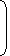=C.P. of dearer – Mean PriceQuantity of dearer Mean price – C.P. of cheaper

We present as under:

 C.P. of a unit quantity of cheaperC.P. of a unit quantity of dearer (c) Mean Price (m) (d) (d – m) (m – c)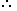(Cheaper quantity) : (Dearer quantity) = (d – m) : (m – c).

4. Suppose a container contains x of liquid from which y units are taken out and replaced by water.
 After n operations, the quantity of pure liquid =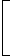x1 – yn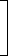units. x
A vessel is filled with liquid, 3 parts of which are water and 5 parts syrup. How much of the mixture must be drawn off and replaced with water so that the mixture may be half water and half syrup?
A.
 1 3
B.
 1 4
C.
 1 5
D.
 1 7

Explanation:

Suppose the vessel initially contains 8 litres of liquid.

Let x litres of this liquid be replaced with water.

 Quantity of water in new mixture =3 – 3x + xlitres 8
 Quantity of syrup in new mixture =5 – 5xlitres 83 – 3x + x=5 – 5x8 8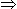5x + 24 = 40 – 5x10x = 16x = 8 . 5
 So, part of the mixture replaced =8 x 1= 1 . 5 8 51
Don't miss out!